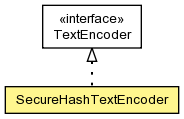## org.jboss.dna.common.text Class SecureHashTextEncoder

```java.lang.Objectorg.jboss.dna.common.text.SecureHashTextEncoder
```
All Implemented Interfaces:
TextEncoder

`public class SecureHashTextEncoderextends Objectimplements TextEncoder`A text encoder that performs a secure hash of the input text and returns that hash as the encoded text. This encoder can be configured to use different secure hash algorithsm and to return a fixed set of characters of the hash.

Constructor Summary
`SecureHashTextEncoder(SecureHash.Algorithm algorithm)`
Create an encoder that uses the supplied algorithm and returns only the supplied number of characters in the hash.
```SecureHashTextEncoder(SecureHash.Algorithm algorithm, int maxLength)```
Create an encoder that uses the supplied algorithm and returns only the supplied number of characters in the hash.

Method Summary
` String` `encode(String text)`
Returns the encoded version of a string.
` SecureHash.Algorithm` `getAlgorithm()`
Return the secure hash algorithm used by this encoder.
` int` `getMaxLength()`
Get the maximum length of the encoded string, or `Integer.MAX_VALUE` if there is no maximum.

Methods inherited from class java.lang.Object
`equals, getClass, hashCode, notify, notifyAll, toString, wait, wait, wait`

Constructor Detail

### SecureHashTextEncoder

`public SecureHashTextEncoder(SecureHash.Algorithm algorithm)`
Create an encoder that uses the supplied algorithm and returns only the supplied number of characters in the hash.

Parameters:
`algorithm` - the algorithm that should be used
Throws:
`IllegalArgumentException` - if the algorithm is null

### SecureHashTextEncoder

```public SecureHashTextEncoder(SecureHash.Algorithm algorithm,
int maxLength)```
Create an encoder that uses the supplied algorithm and returns only the supplied number of characters in the hash.

Parameters:
`algorithm` - the algorithm that should be used
`maxLength` - the maximumLength, or a non-positive number (or `Integer.MAX_VALUE`) if the full hash should be used
Throws:
`IllegalArgumentException` - if the algorithm is null
Method Detail

### getMaxLength

`public int getMaxLength()`
Get the maximum length of the encoded string, or `Integer.MAX_VALUE` if there is no maximum.

Returns:
the maximum encoded string length; always positive

### getAlgorithm

`public SecureHash.Algorithm getAlgorithm()`
Return the secure hash algorithm used by this encoder.

Returns:
the algorithm; never null

### encode

`public String encode(String text)`
Returns the encoded version of a string.

Specified by:
`encode` in interface `TextEncoder`
Parameters:
`text` - the text with characters that are to be encoded.
Returns:
the text with the characters encoded as required, or null if the supplied text is null
`TextEncoder.encode(java.lang.String)`